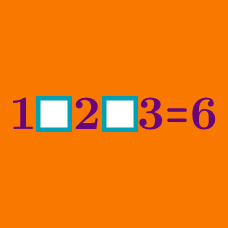Logic

# The 24 Puzzle

$9 \square 1 \square 7 \square 4 = 24$

Which set of operators can be used in the blanks above to make this equation correct? Operators can be placed in the blanks in any order, and any number of parentheses can be used.

Hint: When solving puzzles such as these, it is often helpful to consider different methods that could be used to arrive at your final answer. For example, if the final operator is $\times,$ then we need two numbers whose product is 24. For reference, the nontrivial factors of 24 are 2,3,4,6,8, and 12.

$9 \square 8 \square 3 \square 8 = 24$

Which set of operators can be used in the blanks above to make this equation correct? Operators can be placed in the blanks in any order, and any number of parentheses can be used.

Hint: When solving puzzles such as these, it is often easier to reach the target number using only 2 or three of the required digits. Try to develop strategies for eliminating a number or pair of numbers. This is often done by producing 1, which can be multiplied for no effect, or 0, which can be added for no effect.

$6 \square 1 \square 3 \square 4 = 24$

Which set of operators can be used in the blanks above to make this equation correct? Operators can be placed in the blanks in any order, and any number of parentheses can be used.

Hint: When solving puzzles such as these,remember that the same result can often be produced using different operators. For example, multiplying by 2 is equivalent to dividing by $\frac{1}{2}.$

$7 \square 3 \square 3 \square 7 = 24$

Which set of operators can be used in the blanks above to make this equation correct? Operators can be placed in the blanks in any order, and any number of parentheses can be used.

Hint: When solving puzzles such as these, it is often helpful to try imagining all the possible final steps involving one of the given numbers. For example, if the final step is $\times 5,$ you would need to make $\frac{24}{5}$ with the other 3 numbers. This can be particularly helpful when the solution involves a fractional product.

$6 \square 6 \square 4 \square 1 = 24$

Which set of operators can be used in the blanks above to make this equation correct? Operators can be placed in the blanks in any order, and any number of parentheses can be used.

Hint: When solving puzzles such as these, sometimes it’s not always possible to find factors that multiply nicely to 24. It’s often helpful to look for other, nearby numbers with nice factors, especially ones that can produce 24 when added to or subtracted from the numbers you already have. Numbers that can be helpful include 18, 20, 21, 25, 27, 28, and 30.

×

Problem Loading...

Note Loading...

Set Loading...